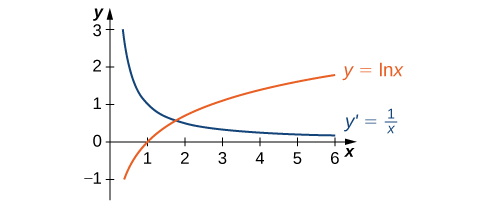# 3.9 Derivatives of exponential and logarithmic functions  (Page 3/6)

 Page 3 / 6

## Proof

If $x>0$ and $y=\text{ln}\phantom{\rule{0.1em}{0ex}}x,$ then ${e}^{y}=x.$ Differentiating both sides of this equation results in the equation

${e}^{y}\frac{dy}{dx}=1.$

Solving for $\frac{dy}{dx}$ yields

$\frac{dy}{dx}=\frac{1}{{e}^{y}}.$

Finally, we substitute $x={e}^{y}$ to obtain

$\frac{dy}{dx}=\frac{1}{x}.$

We may also derive this result by applying the inverse function theorem, as follows. Since $y=g\left(x\right)=\text{ln}\phantom{\rule{0.1em}{0ex}}x$ is the inverse of $f\left(x\right)={e}^{x},$ by applying the inverse function theorem we have

$\frac{dy}{dx}=\frac{1}{{f}^{\prime }\left(g\left(x\right)\right)}=\frac{1}{{e}^{\text{ln}\phantom{\rule{0.1em}{0ex}}x}}=\frac{1}{x}.$

Using this result and applying the chain rule to $h\left(x\right)=\text{ln}\phantom{\rule{0.1em}{0ex}}\left(g\left(x\right)\right)$ yields

${h}^{\prime }\left(x\right)=\frac{1}{g\left(x\right)}{g}^{\prime }\left(x\right).$

The graph of $y=\text{ln}\phantom{\rule{0.1em}{0ex}}x$ and its derivative $\frac{dy}{dx}=\frac{1}{x}$ are shown in [link] .The function y = ln x is increasing on ( 0 , + ∞ ) . Its derivative y ′ = 1 x is greater than zero on ( 0 , + ∞ ) .

## Taking a derivative of a natural logarithm

Find the derivative of $f\left(x\right)=\text{ln}\phantom{\rule{0.1em}{0ex}}\left({x}^{3}+3x-4\right).$

Use [link] directly.

$\begin{array}{ccccc}\hfill {f}^{\prime }\left(x\right)& =\frac{1}{{x}^{3}+3x-4}·\left(3{x}^{2}+3\right)\hfill & & & \text{Use}\phantom{\rule{0.2em}{0ex}}g\left(x\right)={x}^{3}+3x-4\phantom{\rule{0.2em}{0ex}}\text{in}\phantom{\rule{0.2em}{0ex}}{h}^{\prime }\left(x\right)=\frac{1}{g\left(x\right)}{g}^{\prime }\left(x\right).\hfill \\ & =\frac{3{x}^{2}+3}{{x}^{3}+3x-4}\hfill & & & \text{Rewrite.}\hfill \end{array}$

## Using properties of logarithms in a derivative

Find the derivative of $f\left(x\right)=\text{ln}\phantom{\rule{0.1em}{0ex}}\left(\frac{{x}^{2}\text{sin}\phantom{\rule{0.1em}{0ex}}x}{2x+1}\right).$

At first glance, taking this derivative appears rather complicated. However, by using the properties of logarithms prior to finding the derivative, we can make the problem much simpler.

$\begin{array}{cccccc}\hfill f\left(x\right)& =\hfill & \text{ln}\phantom{\rule{0.1em}{0ex}}\left(\frac{{x}^{2}\text{sin}\phantom{\rule{0.1em}{0ex}}x}{2x+1}\right)=2\phantom{\rule{0.1em}{0ex}}\text{ln}\phantom{\rule{0.1em}{0ex}}x+\text{ln}\phantom{\rule{0.1em}{0ex}}\left(\text{sin}\phantom{\rule{0.1em}{0ex}}x\right)-\text{ln}\phantom{\rule{0.1em}{0ex}}\left(2x+1\right)\hfill & & & \text{Apply properties of logarithms.}\hfill \\ \hfill {f}^{\prime }\left(x\right)& =\hfill & \frac{2}{x}+\text{cot}\phantom{\rule{0.1em}{0ex}}x-\frac{2}{2x+1}\hfill & & & \text{Apply sum rule and}\phantom{\rule{0.2em}{0ex}}{h}^{\prime }\left(x\right)=\frac{1}{g\left(x\right)}{g}^{\prime }\left(x\right).\hfill \end{array}$

Differentiate: $f\left(x\right)=\text{ln}{\left(3x+2\right)}^{5}.$

${f}^{\prime }\left(x\right)=\frac{15}{3x+2}$

Now that we can differentiate the natural logarithmic function, we can use this result to find the derivatives of $y=lo{g}_{b}x$ and $y={b}^{x}$ for $b>0,b\ne 1.$

## Derivatives of general exponential and logarithmic functions

Let $b>0,b\ne 1,$ and let $g\left(x\right)$ be a differentiable function.

1. If, $y={\text{log}}_{b}x,$ then
$\frac{dy}{dx}=\frac{1}{x\phantom{\rule{0.1em}{0ex}}\text{ln}\phantom{\rule{0.1em}{0ex}}b}.$

More generally, if $h\left(x\right)={\text{log}}_{b}\left(g\left(x\right)\right),$ then for all values of x for which $g\left(x\right)>0,$
${h}^{\prime }\left(x\right)=\frac{{g}^{\prime }\left(x\right)}{g\left(x\right)\phantom{\rule{0.1em}{0ex}}\text{ln}\phantom{\rule{0.1em}{0ex}}b}.$
2. If $y={b}^{x},$ then
$\frac{dy}{dx}={b}^{x}\text{ln}\phantom{\rule{0.1em}{0ex}}b.$

More generally, if $h\left(x\right)={b}^{g\left(x\right)},$ then
${h}^{\prime }\left(x\right)={b}^{g\left(x\right)}g\text{″}\left(x\right)\phantom{\rule{0.1em}{0ex}}\text{ln}\phantom{\rule{0.1em}{0ex}}b.$

## Proof

If $y={\text{log}}_{b}x,$ then ${b}^{y}=x.$ It follows that $\text{ln}\phantom{\rule{0.1em}{0ex}}\left({b}^{y}\right)=\text{ln}\phantom{\rule{0.2em}{0ex}}x.$ Thus $y\phantom{\rule{0.2em}{0ex}}\text{ln}\phantom{\rule{0.2em}{0ex}}b=\text{ln}\phantom{\rule{0.2em}{0ex}}x.$ Solving for $y,$ we have $y=\frac{\text{ln}\phantom{\rule{0.1em}{0ex}}x}{\text{ln}\phantom{\rule{0.1em}{0ex}}b}.$ Differentiating and keeping in mind that $\text{ln}\phantom{\rule{0.1em}{0ex}}b$ is a constant, we see that

$\frac{dy}{dx}=\frac{1}{x\phantom{\rule{0.1em}{0ex}}\text{ln}\phantom{\rule{0.1em}{0ex}}b}.$

The derivative in [link] now follows from the chain rule.

If $y={b}^{x},$ then $\text{ln}\phantom{\rule{0.2em}{0ex}}y=x\phantom{\rule{0.1em}{0ex}}\text{ln}\phantom{\rule{0.1em}{0ex}}b.$ Using implicit differentiation, again keeping in mind that $\text{ln}\phantom{\rule{0.1em}{0ex}}b$ is constant, it follows that $\frac{1}{y}\phantom{\rule{0.2em}{0ex}}\frac{dy}{dx}=\text{ln}\phantom{\rule{0.1em}{0ex}}b.$ Solving for $\frac{dy}{dx}$ and substituting $y={b}^{x},$ we see that

$\frac{dy}{dx}=y\phantom{\rule{0.1em}{0ex}}\text{ln}\phantom{\rule{0.1em}{0ex}}b={b}^{x}\text{ln}\phantom{\rule{0.1em}{0ex}}b.$

The more general derivative ( [link] ) follows from the chain rule.

## Applying derivative formulas

Find the derivative of $h\left(x\right)=\frac{{3}^{x}}{{3}^{x}+2}.$

Use the quotient rule and [link] .

$\begin{array}{ccccc}\hfill {h}^{\prime }\left(x\right)& =\frac{{3}^{x}\phantom{\rule{0.1em}{0ex}}\text{ln}\phantom{\rule{0.1em}{0ex}}3\left({3}^{x}+2\right)-{3}^{x}\phantom{\rule{0.1em}{0ex}}\text{ln}\phantom{\rule{0.1em}{0ex}}3\left({3}^{x}\right)}{{\left({3}^{x}+2\right)}^{2}}\hfill & & & \text{Apply the quotient rule.}\hfill \\ & =\frac{2·{3}^{x}\phantom{\rule{0.1em}{0ex}}\text{ln}\phantom{\rule{0.1em}{0ex}}3}{{\left({3}^{x}+2\right)}^{2}}\hfill & & & \text{Simplify.}\hfill \end{array}$

## Finding the slope of a tangent line

Find the slope of the line tangent to the graph of $y={\text{log}}_{2}\left(3x+1\right)$ at $x=1.$

To find the slope, we must evaluate $\frac{dy}{dx}$ at $x=1.$ Using [link] , we see that

$\frac{dy}{dx}=\frac{3}{\phantom{\rule{0.1em}{0ex}}\text{ln}\phantom{\rule{0.1em}{0ex}}2\left(3x+1\right)}.$

By evaluating the derivative at $x=1,$ we see that the tangent line has slope

$\frac{dy}{dx}|\begin{array}{l}\\ {}_{x=1}\end{array}=\frac{3}{4\phantom{\rule{0.1em}{0ex}}\text{ln}\phantom{\rule{0.1em}{0ex}}2}=\frac{3}{\phantom{\rule{0.1em}{0ex}}\text{ln}\phantom{\rule{0.1em}{0ex}}16}.$

Find the slope for the line tangent to $y={3}^{x}$ at $x=2.$

$9\phantom{\rule{0.1em}{0ex}}\text{ln}\phantom{\rule{0.1em}{0ex}}\left(3\right)$

## Logarithmic differentiation

At this point, we can take derivatives of functions of the form $y={\left(g\left(x\right)\right)}^{n}$ for certain values of $n,$ as well as functions of the form $y={b}^{g\left(x\right)},$ where $b>0$ and $b\ne 1.$ Unfortunately, we still do not know the derivatives of functions such as $y={x}^{x}$ or $y={x}^{\pi }.$ These functions require a technique called logarithmic differentiation    , which allows us to differentiate any function of the form $h\left(x\right)=g{\left(x\right)}^{f\left(x\right)}.$ It can also be used to convert a very complex differentiation problem into a simpler one, such as finding the derivative of $y=\frac{x\sqrt{2x+1}}{{e}^{x}{\text{sin}}^{3}x}.$ We outline this technique in the following problem-solving strategy.

#### Questions & Answers

Find the derivative of g(x)=−3.
Abdullah Reply
any genius online ? I need help!!
Guzorochi Reply
how can i help you?
Pina
need to learn polynomial
Zakariya
i will teach...
nandu
I'm waiting
Zakariya
plz help me in question
Abish
evaluate the following computation (x³-8/x-2)
Murtala Reply
teach me how to solve the first law of calculus.
Uncle Reply
what is differentiation
Ibrahim Reply
f(x) = x-2 g(x) = 3x + 5 fog(x)? f(x)/g(x)
Naufal Reply
fog(x)= f(g(x)) = x-2 = 3x+5-2 = 3x+3 f(x)/g(x)= x-2/3x+5
diron
pweding paturo nsa calculus?
jimmy
how to use fundamental theorem to solve exponential
JULIA Reply
find the bounded area of the parabola y^2=4x and y=16x
Omar Reply
what is absolute value means?
Geo Reply
Chicken nuggets
Hugh
🐔
MM
🐔🦃 nuggets
MM
(mathematics) For a complex number a+bi, the principal square root of the sum of the squares of its real and imaginary parts, √a2+b2 . Denoted by | |. The absolute value |x| of a real number x is √x2 , which is equal to x if x is non-negative, and −x if x is negative.
Ismael
find integration of loge x
Game Reply
find the volume of a solid about the y-axis, x=0, x=1, y=0, y=7+x^3
Godwin Reply
how does this work
Brad Reply
Can calculus give the answers as same as other methods give in basic classes while solving the numericals?
Cosmos Reply
log tan (x/4+x/2)
Rohan
please answer
Rohan
y=(x^2 + 3x).(eipix)
Claudia
is this a answer
Ismael
A Function F(X)=Sinx+cosx is odd or even?
WIZARD Reply
neither
David
Neither
Lovuyiso
f(x)=1/1+x^2 |=[-3,1]
Yuliana Reply
apa itu?
fauzi

### Read also:

#### Get Jobilize Job Search Mobile App in your pocket Now!

Source:  OpenStax, Calculus volume 1. OpenStax CNX. Feb 05, 2016 Download for free at http://cnx.org/content/col11964/1.2
Google Play and the Google Play logo are trademarks of Google Inc.

Notification Switch

Would you like to follow the 'Calculus volume 1' conversation and receive update notifications?By OpenStaxBy Dindin SecretoBy OpenStaxBy John GabrieliBy OpenStaxBy Katy PrattBy Stephen VoronBy OpenStaxBy Jonathan LongBy IES Portal